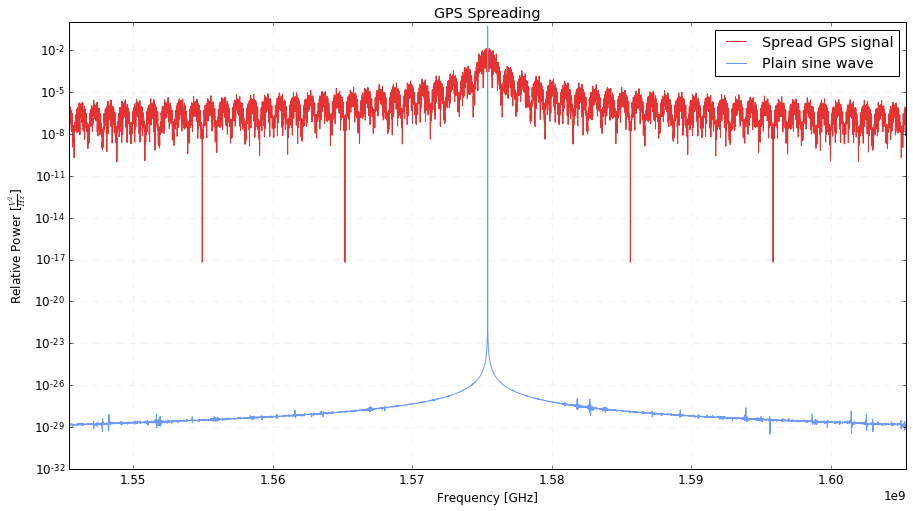# natronics on GitHub

## GPS Spreading Properties – November 2014

In the previous posts I talked about the basic look of GPS signals and then went into detail about the special sequences used in actual GPS satellites. But why does GPS use a psudo random number? And what about the sequence is useful?

The general technique used in GPS is called code division multiple access (CDMA). It’s an example of spread-spectrum radio techniques, where you actully make the signal harder to see in the noise, but in a reversable manor that, after processing, eventually make the signal easier to receive. This is a little counterintuitive, so bare with me.

The GPS PRNs are a kind of “spreading code”. Because there are sharp corners in the signal (at every point the phase jumps 180°) the amount of power is spread across a large number of frequencies. Remember from looking at square waves that sharp corners in the time domain are made of a composite of an infinite squence of frequencies. And worse, the phase changes are randomly distributed by the PRN. The final effect is to make the GPS signal look almost like noise! The radio power is divided up across the spectrum instead of being entirely in the carrier wave.

## Sample an Example

To create an example we’ll create a PRN for satellite 24 (pulled out of a hat). Then we’ll make a GPS signal by applying that PRN to a sine wave. Then we’ll sample that signal at 100 GHz to end up with a descrete sample of a ‘real’ GPS signal!

Now that we have a sample, we can compute power spectrums. We’ll do this for both a plain sine wave and for the GPS signal.

Now we can chart the two spectrums and compare them.Whats going on here? The sine wave has very little power at any fequency except at its exact frequency. So the ‘floor’ is very low, and there is very, very strong peak. Meanwhile after adding in the PRN the power is spread across the entire spectrum. So there is little power left at the main frequency, but there is a lot every where else.

There are other properties like autocorrelation and orthogonality that are important too. But that’s for another day.